# Electric Fields Electric fields are created by electric

• Slides: 6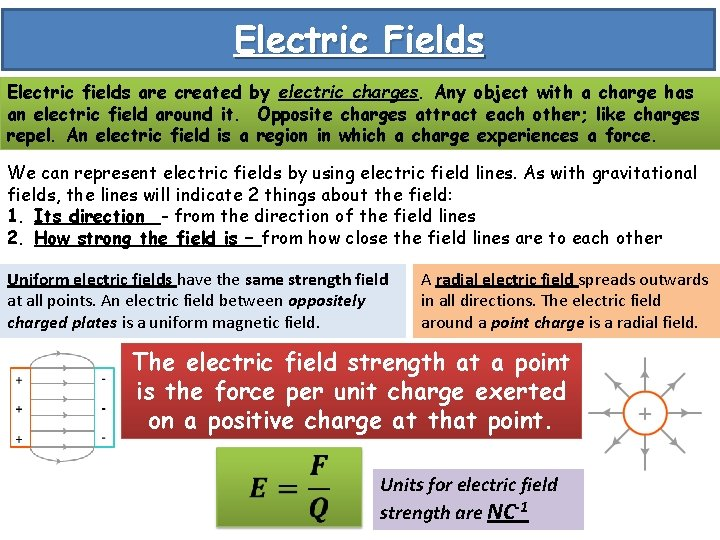Electric Fields Electric fields are created by electric charges. Any object with a charge has an electric field around it. Opposite charges attract each other; like charges repel. An electric field is a region in which a charge experiences a force. We can represent electric fields by using electric field lines. As with gravitational fields, the lines will indicate 2 things about the field: 1. Its direction - from the direction of the field lines 2. How strong the field is – from how close the field lines are to each other Uniform electric fields have the same strength field at all points. An electric field between oppositely charged plates is a uniform magnetic field. A radial electric field spreads outwards in all directions. The electric field around a point charge is a radial field. The electric field strength at a point is the force per unit charge exerted on a positive charge at that point. Units for electric field strength are NC-1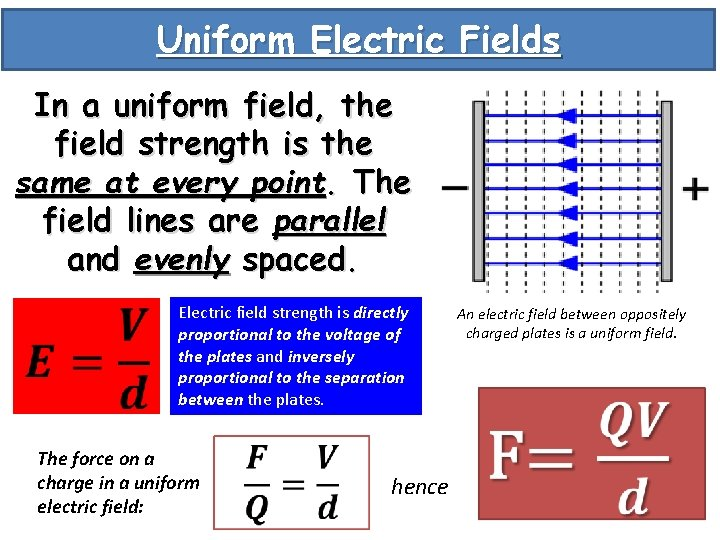Uniform Electric Fields In a uniform field, the field strength is the same at every point. The field lines are parallel and evenly spaced. Electric field strength is directly proportional to the voltage of the plates and inversely proportional to the separation between the plates. The force on a charge in a uniform electric field: hence An electric field between oppositely charged plates is a uniform field.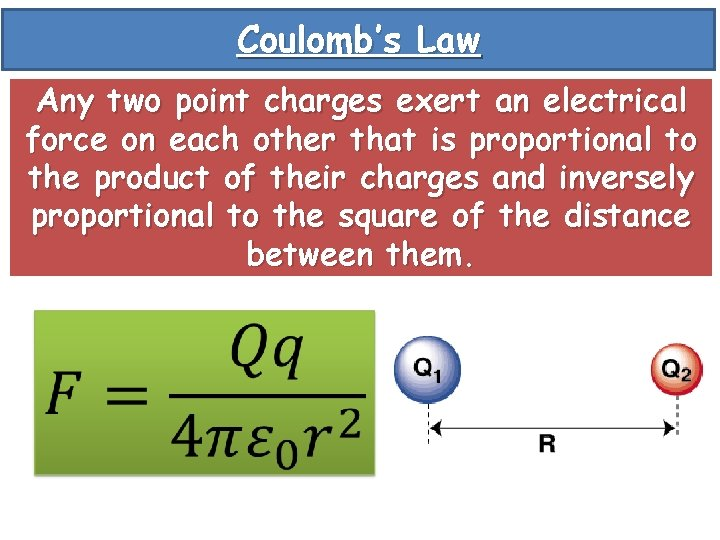Coulomb’s Law Any two point charges exert an electrical force on each other that is proportional to the product of their charges and inversely proportional to the square of the distance between them.Radial Electric Fields Radial magnetic fields spread outwards in all directions. For a radial field, the electric field strength depends on the distance r from the point charge Q. There is an inverse square relationship – field strength decreases as you get further from the point charge. Electric field strength for a radial field: This is shown by the fields lines: they get further apart from each other as you move away from the point charge.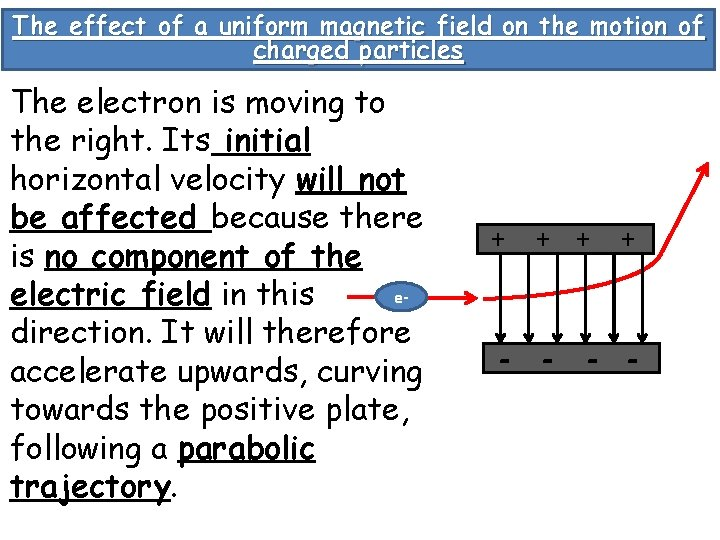The effect of a uniform magnetic field on the motion of charged particles The electron is moving to the right. Its initial horizontal velocity will not be affected because there is no component of the eelectric field in this direction. It will therefore accelerate upwards, curving towards the positive plate, following a parabolic trajectory. + + - -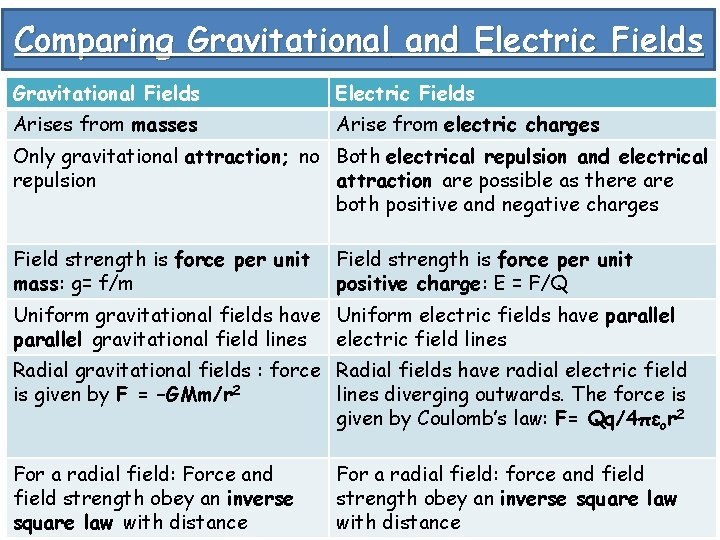Comparing Gravitational and Electric Fields Gravitational Fields Electric Fields Arises from masses Arise from electric charges Only gravitational attraction; no Both electrical repulsion and electrical repulsion attraction are possible as there are both positive and negative charges Field strength is force per unit mass: g= f/m Field strength is force per unit positive charge: E = F/Q Uniform gravitational fields have Uniform electric fields have parallel gravitational field lines electric field lines Radial gravitational fields : force Radial fields have radial electric field is given by F = –GMm/r 2 lines diverging outwards. The force is given by Coulomb’s law: F= Qq/4πεor 2 For a radial field: Force and field strength obey an inverse square law with distance For a radial field: force and field strength obey an inverse square law with distance